# Quiz 12: Aggregate Demand and Aggregate Supply

Business

a decrease of \$200; a decrease of \$40; 5. Feedback: Consider the following example.  Refer to FigurE1a and 1b in the Appendix. Assume that Q 1 iS300, Q 2 iS200, Q 3 iS100, P3 iS120, P2 iS100, and P1 is 80. If the price level increases from P1 to P3 in graph (b), in what direction and by how much will real GDP change If the slopes of the AE lines in graph (a) are.8 and equal to the MPC, in what direction will the aggregate expenditures schedule in graph (a) need to shift to produce the previously determined change in real GDP What is the size of the multiplier in this example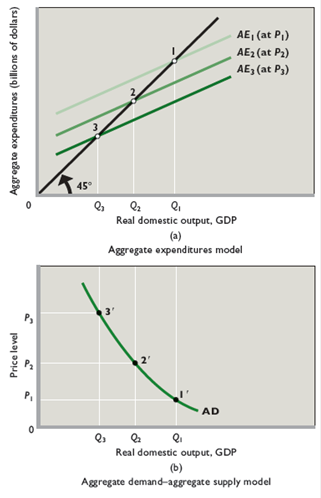If the price level increases from P1 to P3 in graph (b), in what direction and by how much will real GDP change Real GDP will fall (movement along the AD schedule and a shift downward of the AE schedule). The decrease in real GDP will bE200 (Q 1 - Q 3 = 300 - 100 = 200). If the slopes of the AE lines in graph (a) are.8 and equal to the MPC, in what direction will the aggregate expenditures schedule in graph (a) need to shift to produce the previously determined change in real GDP What is the size of the multiplier in this example The first step is to determine the multiplier for this problem. Given the MPC is 0.8 the multiplier is 5 (= 1/(1-MPC) = 1/(1-0.8) = 1/0.2 = 5). Since the multiplier is 5, we can find by how much the aggregate expenditure curve must change. Real GDP decreases, which implies the AE schedule must fall (to AE3 at P3 ). The AE curve must fall by \$40 since the total decrease in output is \$200. To see this, note that a decrease in AE by \$40 with a multiplier of 5 is \$200 (= 5 x \$40). Change in real GDP = multiplier x change in AE Rearranging this equation, Change in AE = change in real GDP/multiplier Using the values above, the change in AE equals -\$200/5 = -\$40, or we can express the negative as a decrease of \$40 as above.

A change in the price level does not shift the aggregate demand curve.  It simply represents a movement along the curve, because there is an inverse relationship between the price level and aggregate quantity demanded. However, a change in the price level will shift the aggregate expenditures curve, which responds to the wealth, interest-rate, and foreign purchases effects occurring with a change in price level.  When the price level declines, aggregate expenditures will rise, and when the price level rises, aggregate expenditures will fall.  The aggregate expenditures model assumes a constant price level, so it is expressed in "real" terms.  Appendix FigureS1 and 2 graphically illustrates the relationship between the two models.

Aggregate Demand Aggregate demand refers to the quantity of all the products and services that is demand within the economy during the period of a financial year. It includes the amounts of products and services that the population is willing purchase at all price levels. Real Interest Rate The real interest rate is the interest rate that an investor gets after subtracting the rate of inflation. According to the Fisher equation, Real interest rate can be calculated by subtracting the inflation rate with the nominal interest rate. Initial Change in aggregate demand: Fall in the household wealth: Due to a fall in the household wealth by 5%, the consumer spending will decline by \$5 billion per 1 percent. Therefore, the decline consumer spending due to declining house values is;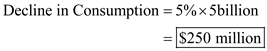Thus, the consumption part of aggregate demand declines by \$250 million. Fall in real interest rate: On the other hand, interest rates declines by 2%, furthermore, it is given that the investment spending increases by \$20 billion for every percentage decline in the real interest rate. Therefore, the increase in investment spending due to fall in real interest rates is;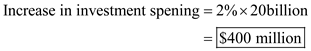Furthermore, the net effect of an increase in investment spending and a decrease in consumer spending on the aggregate demand is shown below;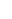Thus, the aggregate demand curve shifts towards right by \$150 million initially; however, due to multiplier effect the aggregate demand curve shifts further, the shift in aggregate demand due to multiplier effect is shown below;Thus, due to a decline in hose values and a decline in real interest rates the aggregate demand curve initially shifts rightward by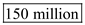at each price level; and eventually, rightward by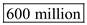due to multiplier effect.